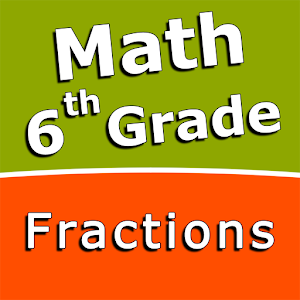Română

În fiecare zi oferim GRATUIT aplicații și jocuri Android licențiate pe care altfel ar trebui să le cumpărați.\$2.99
EXPIRAT

# Android Giveaway of the Day - Fractions and mixed numbers - 6th grade math

Add fractions and mixed numbers with like denominators.
\$2.99 EXPIRAT
Părerea utilizatorilor: 0 (0%) 0 (0%)

This giveaway offer has been expired. Fractions and mixed numbers - 6th grade math is now available on the regular basis.

Intuitive interface powered by handwriting input and a choice of three fun and engaging mini games in addition to a regular math trainer mode make our app stand out from the crowd of generic math learning apps.

With Fractions and mixed numbers - 6th grade math you can practice and improve the following math skills:
- Write fractions in lowest terms
- Add fractions and mixed numbers with like denominators
- Add fractions and mixed numbers with unlike denominators
- Subtract fractions and mixed numbers with like denominators
- Subtract fractions and mixed numbers with unlike denominators
- Multiply fractions and mixed numbers
- Multiply mixed numbers and whole numbers
- Divide fractions and mixed numbers
- Divide mixed numbers by whole numbers
- Convert fractions and mixed numbers to decimals

Sergey Malugin

Educational

### Versiune:

Varies with device

### Dimensiune:

Varies with device

Everyone

### Compatibilitate:

Varies with device

## Comentarii la Fractions and mixed numbers - 6th grade math

Thank you for voting!